# Themes, topics - math word problems

1. Free fall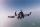Lloyd fall from height 7 m. Calculate the speed he hit the ground when falling with acceleration g = 9.81 m/s2
2. TractorsFields go plow two tractors with various performance. The first tractor plow whole field in 11 hours, the second tractor plow whole field 19 hours longer. How long take plow whole field with two tractors?
3. Resistors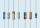Two resistors connected in series give the resulting resistance 216Ω and 48Ω in parallel. Determine the resistance of these resistors.
4. Wind drift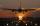The plane flies at 860 km/h, passing distance 3000 kilometers with the wind and once again against the wind for 6 h 59 min. What is the wind speed?Passenger car and an ambulance come to the rectangular crossroad, the ambulance left. Passenger car at speed 39 km/h and ambulance at 41 km/h. Calculate such a relative speed of the ambulance move to the car.
6. Acid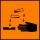The barrel is 199 litres of 38% acid. How many percent acid occurs when 61 litres revoke and replace it with the same amount of water?
7. Trolleybus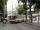Trolleybus line No. 206 measured 24 km. If the trolleybus goes faster by 5 km/h, the way there and back would is shorter by 33 minutes. Calculate the trolleybus speed and how much time it takes a return trip.
8. Rotation of the EarthCalculate the circumferential speed of the Earth's surface at a latitude of 61°​​. Consider a globe with a radius of 6378 km.

We apologize, but in this category are not a lot of examples.
Do you have an interesting mathematical word problem that you can't solve it? Enter it, and we can try to solve it.

To this e-mail address, we will reply solution; solved examples are also published here. Please enter the e-mail correctly and check whether you don't have a full mailbox.

Please do not submit problems from current active competitions such as Mathematical Olympiad, correspondence seminars etc...